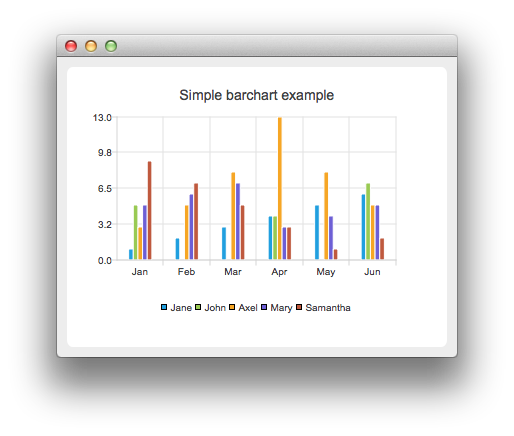BarChart Example

The example shows how to create a bar chart.

BarChart shows the data in sets as separate bars, which are in categories.Running the Example

To run the example from Qt Creator, open the Welcome mode and select the example from Examples. For more information, visit Building and Running an Example.

Creating Bar Charts

The barsets are used in the same way in all barcharts. To illustrate the difference between various barcharts, we use the same data in the examples. Data that the barchart visualizes, is defined by the QBarSet instances. Here we create the sets and append data to them. The data is appended here with the << operator. Alternatively, the append method could be used.

QBarSet *set0 = new QBarSet("Jane");
QBarSet *set1 = new QBarSet("John");
QBarSet *set2 = new QBarSet("Axel");
QBarSet *set3 = new QBarSet("Mary");
QBarSet *set4 = new QBarSet("Samantha");

*set0 << 1 << 2 << 3 << 4 << 5 << 6;
*set1 << 5 << 0 << 0 << 4 << 0 << 7;
*set2 << 3 << 5 << 8 << 13 << 8 << 5;
*set3 << 5 << 6 << 7 << 3 << 4 << 5;
*set4 << 9 << 7 << 5 << 3 << 1 << 2;

We create the series and append the barsets to it. The series takes ownership of the barsets. The series groups the data from sets to categories. First values of each set are grouped together at first category second value to second category and so on.

QBarSeries *series = new QBarSeries();
series->append(set0);
series->append(set1);
series->append(set2);
series->append(set3);
series->append(set4);

Here we create the chart object and add the series to it. We set the title for chart with setTitle and then turn on animations of the series by calling setAnimationOptions(QChart::SeriesAnimations)

QChart *chart = new QChart();
chart->setTitle("Simple barchart example");
chart->setAnimationOptions(QChart::SeriesAnimations);

To have categories displayed on axis, we need to create a QBarCategoryAxis for that. Here we create a category axis with a list of categories and set it to be aligned to the bottom, acting as the x-axis, and attach it to the series. The chart takes ownership of the axis. For y-axis we use a value axis, aligned to the left-hand side.

QStringList categories;
categories << "Jan" << "Feb" << "Mar" << "Apr" << "May" << "Jun";
QBarCategoryAxis *axisX = new QBarCategoryAxis();
axisX->append(categories);
series->attachAxis(axisX);

QValueAxis *axisY = new QValueAxis();
axisY->setRange(0,15);
series->attachAxis(axisY);

We also want to show the legend. To do that, we get the legend pointer from the chart and set it to visible. We also place the legend to the bottom of the chart by setting its alignment to Qt::AlignBottom.

chart->legend()->setVisible(true);
chart->legend()->setAlignment(Qt::AlignBottom);

Finally we add the chart onto a view. We also turn on the antialiasing for the chartView.

QChartView *chartView = new QChartView(chart);
chartView->setRenderHint(QPainter::Antialiasing);

The chart is ready to be shown. We set the chart to be central widget of the window. We also set the size for the chart window and show it.

QMainWindow window;
window.setCentralWidget(chartView);
window.resize(420, 300);
window.show();

Example project @ code.qt.io